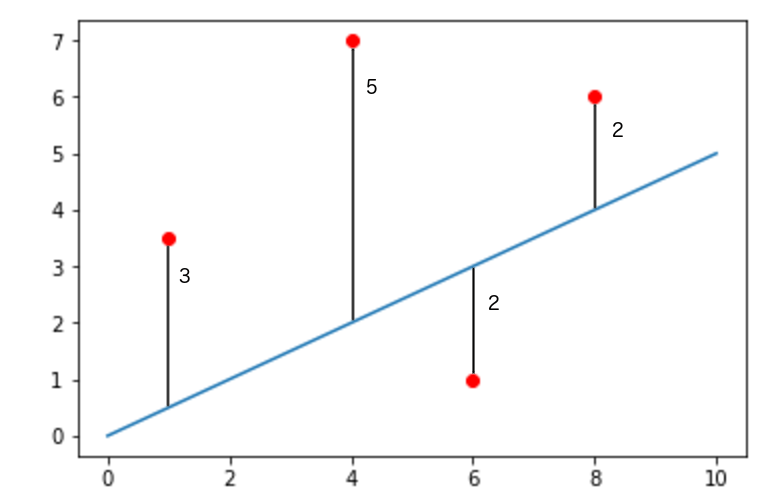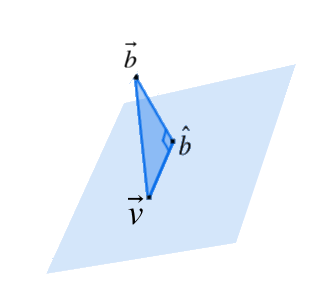### Machine Learning

Note: This quiz requires some knowledge of linear algebra.

Statistical tools are extremely powerful tools when analyzing data, but they are not the only tools. Linear algebra, for instance, often presents a more intuitive way to view best-fit lines that is easier to generalize.

The best-fit line given by the equation $y - \overline{y} = \frac{rSD_y}{SD_x}(x-\overline{x})$ is actually known as the least squares regression line, which means that if we sum the square of the vertical distance from each data point to the best-fit line, the result will be less than it would be for any other line.

This definition allows us to define an error function for any given line $y = mx+b$ which outputs the sum of squared errors, or the sum of the square of each point’s vertical distance from a line. This quantity is often abbreviated as SSE. We use this as a measure of how much a regression line deviates from the actual data set. The least squares regression line is the line for which the error function is at its minimum value.

If we put this in mathematical terms we find the formula, $SSE = \sum_{i=1}^{n} (y_i - mx_i - b)^2$ Now, we can think of our best-fit line as the line with values of $m$ and $b$ which minimize the error function. This is extremely useful because it gives us a concrete set of criteria for our best-fit line which we can expand on to suit our needs. For now, though, we will just work with our new definition in an unaltered state.

# Linear Algebra in Linear Regression

There are several points in the scatter plot shown as well as a best-fit line candidate. We have shown the vertical distance from each point to the line. What is the SSE?# Linear Algebra in Linear Regression

One benefit of our new definition is that it gives us an alternative method for calculating our best-fit line, one which uses linear algebra techniques.

Say we have a data set containing $n$ points: $(x_1, y_1), (x_2, y_2),\ldots, (x_n, y_n).$ We need to derive a formula which can give us the least squares regression line for our data set, so a good first step is to put our variables into linear algebra terms.

First, we realize that since every line can be represented by the equation $y = mx + b,$ we can also represent every line with a single, two-dimensional vector that stores the line's slope and y-intercept: $\vec{x} = \begin{bmatrix} m \\ b \\ \end{bmatrix}.$ Now, we define an $n \times 2$ matrix $A.$ For $1 \leq i \leq n,$ the $i^\text{th}$ row of $A$ will contain $x_i$ in the first column and 1 in the second: $A = \begin{bmatrix} x_1 & 1\\x_2 & 1 \\ \vdots & \vdots \\ x_n & 1\\ \end{bmatrix}.$ This definition may appear somewhat arbitrary, but it becomes useful when we multiply $A$ by a vector representing a line. For any vector $\vec{x} = \begin{bmatrix} m \\ b \\ \end{bmatrix},$ the vector given by $A\vec{x}$ will contain the $y$-values the line represented by $\vec{x}$ will predict for each $x$-value in our data set. In other words, calculating $A\vec{x}$ is like feeding the $x$-value from each point into the function represented by $\vec{x}.$

As a result, if we define a new vector $\vec{b}$ so that its $i^\text{th}$ element will be $y_i$ from our data set, we can find the vertical distance between each point and the $y$-value predicted for it by subtracting $\vec{b}$ from $A\vec{x}.$

We can now find the SSE by individually squaring the values inside $A\vec{x}-\vec{b}$ and adding them together. Interestingly, this process is equivalent to squaring the length of $A\vec{x}-\vec{b},$ so the SSE is equal to the squared distance in $n$-dimensional space between $A\vec{x}$ and $\vec{b}.$

In other words, $SSE = \Big|\Big|A\vec{x}-\vec{b}\Big|\Big|^2.$

# Linear Algebra in Linear Regression

Now, let’s say we have a data set of just three points: $(1, 0), (3, 4), (2, 3).$ Verify the previous findings by using our new formula to calculate the SSE of line $y = 3x + 2.$

# Linear Algebra in Linear Regression

Finally, we are in a position to use linear algebra techniques to find a formula for our best-fit line.

First, we must realize that when we are minimizing the SSE, we are actually minimizing $||A\vec{x}-\vec{b}||^2.$ This is equivalent to minimizing the distance between $A\vec{x}$ and $\vec{b}$ since minimizing a squared positive value will also minimize the value itself.

So, what we need to find is the vector $\vec{x}$ for which $A\vec{x}$ is as close as possible to $\vec{b}$. To rephrase this question, we need the vector in $A$’s column space which is closest to $\vec{b}$.

# Linear Algebra in Linear Regression

Suppose we have a column space in $\mathbf{R}^3$, $W$, a vector $\vec{b}$, and $A\vec{x}$, the point closest to $\vec{b}$ on $W.$

It would make sense if $A\vec{x}$ was equal to $\hat{b}$, the projection of $\vec{b}$ onto $W.$ It turns out there is a simple proof that this is true, but let's try to find an intuitive reason.

If you shine a light source directly above $\vec{b}$ and the plane representing $W,$ then $\vec{b}$ casts a shadow onto the plane. This shadow corresponds to the projection of $\vec{b}$ onto $W,$ which we call $\hat{b}.$ The tip of the shadow lies on the plane and some careful thought should convince you that no point can be closer to the tip of $\vec{b}$ and still be on the plane $W.$

We begin by picking an arbitrary point on $W$, $\vec{v}$. To get from $\vec{v}$ to $\vec{b}$, we can first travel to $\hat{b}$, and then travel perpendicularly from $\hat{b}$ to $\vec{b}$. $\hat{b}$ is given by drawing a perpendicular line from $\vec{b}$ to $W,$ so Pythagorean’s theorem shows us that $||\vec{v}-\vec{b}||^2 = ||\hat{b}-\vec{v}||^2 + ||\vec{b}-\hat{b}||^2.$ This is depicted in the picture below:This means that no point on $W$ can be closer to $\vec{b}$ than $\hat{b}$, and that $A\vec{x}$ must equal $\hat{b}$. In other words, if we draw a perpendicular line from $\vec{b}$ to $W,$ the point where it intersects with $W$ will be the point on $W$ closest to $\vec{b}$.

# Linear Algebra in Linear Regression

Derive an equation to solve for the optimum $\vec{x}$. Use the fact that $A\vec{x}$ is perpendicular to $\vec{b}-A\vec{x}$ when $\vec{x}$ is the closest to a solution for $A\vec{x} = \vec{b}$.

×